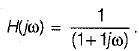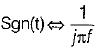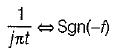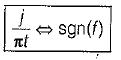Courses

# Test: Continuous Time Fourier Transformer (CTFT)- 1

## 10 Questions MCQ Test Topicwise Question Bank for Electronics Engineering | Test: Continuous Time Fourier Transformer (CTFT)- 1

Description
This mock test of Test: Continuous Time Fourier Transformer (CTFT)- 1 for Electronics and Communication Engineering (ECE) helps you for every Electronics and Communication Engineering (ECE) entrance exam. This contains 10 Multiple Choice Questions for Electronics and Communication Engineering (ECE) Test: Continuous Time Fourier Transformer (CTFT)- 1 (mcq) to study with solutions a complete question bank. The solved questions answers in this Test: Continuous Time Fourier Transformer (CTFT)- 1 quiz give you a good mix of easy questions and tough questions. Electronics and Communication Engineering (ECE) students definitely take this Test: Continuous Time Fourier Transformer (CTFT)- 1 exercise for a better result in the exam. You can find other Test: Continuous Time Fourier Transformer (CTFT)- 1 extra questions, long questions & short questions for Electronics and Communication Engineering (ECE) on EduRev as well by searching above.
QUESTION: 1

Solution:
QUESTION: 2

Solution:
QUESTION: 3

### The fourier transform of real value time signal has

Solution:
QUESTION: 4

The signal x(t) is a real and odd function of ‘t’ the x(ω) is

Solution:
QUESTION: 5

A linear system has the transfer functionWhen it is subjected to an input white noise process with a constant spectral dencity ‘A’ the spectral density of the output will be

Solution:
QUESTION: 6

The auto -correlation of a wide sense stationary random process is given by e-2|τ|. The peak valve of the spectral density is

Solution:
QUESTION: 7

Which one of the following is the correct fourier transform of the unit step signal?
u(t) = 1 for t > 0
= 0 for t < 0

Solution:
QUESTION: 8

The fourier transform of a conjugate symmetric function is always

Solution:
QUESTION: 9

The inverse fourier transform of sgn(f)

Solution:then∴∴QUESTION: 10

Fourier transform f(jω) of an arbitrary signal has the property

Solution: